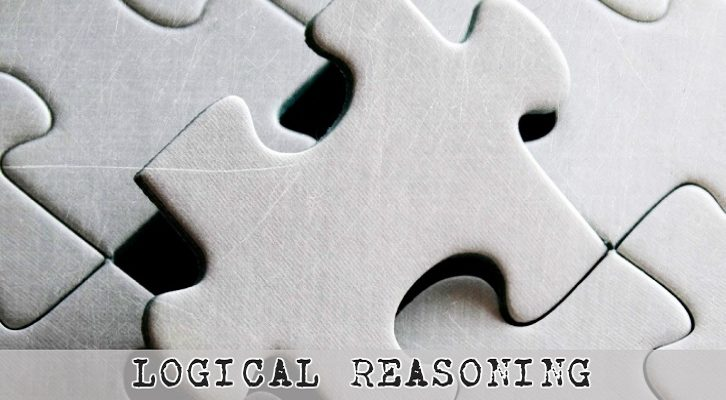# Solution to Speed Time Distance LR question

Have given below the solution to the question on Speed Time Distance LR .

The question is as follows

Station X of length 900 meters has two station masters A and B. But as the station is not a busy one, they are mostly jobless and decide to conduct an experiment. They stand at either end of the station and decide to note the exact time when trains cross the stationmasters. They synchronize their watches and proceed to either end of the station. Two trains P and Q go past the station (neither train stops here), and after having taken down their readings, the station masters sit down to have a chat

1. A: Train P entered the station at exactly 8:00:00
2. B: Train Q entered the station at exactly 8:00:10 (10 seconds past 8)
3. A: The last carriage of train P crossed me by at 8:00:20, and precisely two seconds after this, the engines of the two trains went past each other. (Engines are at the front of the train)
4. B: The last carriage of train Q crossed me 22 seconds after the engine of P went past me.
5. A: After the last carriage of train P crossed by me, it took 35 seconds for the engine of train Q to cross me.
6. B: I got bored and I came here.

Now, let us try to jot down the points in a slightly different format and see if we can make any inferences. Let us assume speed of train P = p, speed of train Q = q, length of train P = L, length of train Q = M

Take statements 1 and 3

Train P crosses the stationmaster A entirely in 20 seconds (enters station at 8:00:00 and last carriage passes at 8:00:20) => Length of train = 20p => L = 20p

Statement 5 tells us that engine of train Q crosses station master A at 8:00:55. Train Q enters the station at 8:00:10, so the train takes 45 seconds to cross the entire station. Or, it takes 45 seconds to travel 900 meters => Speed to train Q, q = 20m/s.

Statement 3 tells us that the two trains cross each other at 8:00:22. This implies train P has traveled for 22 seconds since entering the station and train Q has traveled for 12 seconds since entering the station before they cross each other. The cumulative distance traveled by the two trains should be equal to the length of the station = 900m.

=> 900 = 22p + 12q

q = 20m/s => p = 30m/s.

So, train P_ takes 900/p = 30 seconds to cross the station. So, engine of train P will cross stationmaster B at 8:00:30.

Statement 4 states that the last carriage of Q went past 22 seconds after the engine of P went by. Or, the last carriage of Q went by at 8:00:52.

Engine of train Q went by at 8:00:10, last carriage went by at 8:00:52, or train Q took 42 seconds to cross station master B. Train Q travels at 20m/s. => Length of train Q = 840m

Now, to the questions

1. What is the length of train Q?

Train Q is 840m long

1. At what time do the rear ends of the two trains cross each other?

The engines cross each other at 8:00:22. The relative speed of the two trains = 20+30 = 50m/s. The relative distance traveled by two trains from the time the engines cross each other to the times the rear ends cross each other = Sum of the two lengths = 600 + 840 =1440. Time taken = 1440/50 = 28.8 seconds past 8:00:22, or at 8:00:50.8 seconds.

1. How far from station master A do the rear ends of the two trains cross each other?

At 8:00:50.8, P would have traveled 50.8 * 30m/s post entering the station. Or, train P would have traveled 1524m. The rear end of train P would be at a point 1524-600m = 924m from stationmaster A (or 24 meters from stationmaster B and outside the station)

1. You are told that the two trains enter the station at the same times mentioned and the length of the two trains are unchanged. Furthermore, train P continues to travel at the same speed (as computed above). At what minimum speed should train Q travel such that the rear ends of the two trains cross each other at a point within the length of the platform?

Rear end of train P crosses the station completely at 8:00:50. (Train P takes 30 seconds to travel the station and 20 seconds to travel a distance equal to its length). Train Q should have traveled 840m by this time. => Train Q should travel 840 within 40 seconds.

Or, minimum speed of Q = 840/40 = 21m/s.